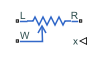# Potentiometer

Rotary or linear-travel potentiometer controlled by physical signal

• Library:
• Simscape / Electrical / Passive

•## Description

The Potentiometer block represents a rotary or linear-travel potentiometer, with the wiper position controlled by the input physical signal.

If the potentiometer resistance changes linearly based on wiper position, then the resistance between the wiper position and port L is:

`${R}_{WL}=\frac{{R}_{0}-2r}{{x}_{\mathrm{max}}-{x}_{\mathrm{min}}}\left(x-{x}_{\mathrm{min}}\right)+r$`

where

• RWL is the resistance between the wiper position and port L.

• R0 is the total resistance between ports L and R.

• x is the wiper position.

• xmin is the value of the wiper position when the wiper is at port L.

• xmax is the value of the wiper position when the wiper is at port R.

• r is the residual resistance. This is the resistance between the wiper and port L when x is equal to xmin. This is also the resistance between the wiper and port R when x is equal to xmax.

If you specify `Logarithmic` for the potentiometer resistance parameter, then the resistance between the wiper position and port L is:

where A and λ are chosen such that RWL at xmax is R0 - r, and RWL at x = (xmax + xmin) / 2 is equal to Rav, the resistance when the wiper is centered.

Note

Potentiometers widely described as LOG or logarithmic taper are, in fact, exponential taper. That is, the gradient of the resistance between wiper and left-hand port increases as the resistance increases. The Potentiometer block implements this behavior.

For both linear and logarithmic tapers, the resistance between the wiper position and port R is:

`${R}_{WR}={R}_{0}-{R}_{WL}$`

where

• RWR is the resistance between the wiper position and port R.

• R0 is the total resistance between ports L and R.

• RWL is the resistance between the wiper position and port L.

## Ports

### Input

expand all

Physical signal input port controlling the wiper position.

### Conserving

expand all

Electrical conserving port associated with the potentiometer left pin.

Electrical conserving port associated with the potentiometer right pin.

#### Dependencies

To enable this port, set to ```Three: left, right, and wiper```.

Electrical conserving port associated with the potentiometer wiper pin.

## Parameters

expand all

Whether to enable the right end port of the potentiometer, R.

Resistance between port L and port R when port W is open-circuit.

#### Dependencies

To enable this parameter, set to ```Three: left, right, and wiper```.

Resistance measured between the wiper and the adjacent end port (L or R) when the wiper is at the end port. You can use this parameter to represent the inability of the wiper to reach the end of the track due to the mechanical stop. This value must be greater than or equal to zero. A typical value is `5e-3` times the total resistance.

#### Dependencies

To enable this parameter, set to ```Three: left, right, and wiper```.

Value of the input physical signal at port x that corresponds to the wiper being located at port L.

#### Dependencies

To enable this parameter, set to ```Three: left, right, and wiper```.

Value of the input physical signal at port x that corresponds to the wiper being located at port `R`.

#### Dependencies

To enable this parameter, set to ```Three: left, right, and wiper```.

Minimum permissible resistance measured between the wiper and the left port, L, when the wiper is at the left port. The value of this parameter must be greater than `0` and lower than the value of the Maximum resistance parameter.

#### Dependencies

To enable this parameter, set to ```Two: left and wiper```.

Maximum permissible resistance measured between the wiper and the left port, L. The value of this parameter must be greater than `0` and greater than the value of the Minimum resistance parameter.

#### Dependencies

To enable this parameter, set to ```Two: left and wiper```.

Value of the input physical signal at port x that corresponds to the wiper being located at port L. The default value is `0`.

#### Dependencies

To enable this parameter, set to ```Two: left and wiper```.

Value of the input physical signal at port x that corresponds to the wiper being located the furthest from port L.

#### Dependencies

To enable this parameter, set to ```Two: left and wiper```.

Resistance taper behavior of the potentiometer.

Note

`Linear` and `Logarithmic` options do not model the same taper. You cannot parameterize the logarithmic potentiometer as a linear potentiometer, because the logarithmic taper is nonlinear.

If you set this parameter to `Linear`, this plot shows the relationship between the wiper control position and the resistance between L and W ports.If you set this parameter to `Logarithmic`, this plot shows the relationship between the wiper control position and the resistance between L and W ports.If you set the Resistance gradient parameter to `Higher at L`, this parameter is the resistance between port L and port W when the wiper is centered. Otherwise, if you set Resistance gradient to `Higher at R`, this parameter is the resistance between port R and port W when the wiper is centered. Because the resistance taper is exponential in shape, the value of the Resistance when centered parameter must be less than half of the Total resistance parameter value.

#### Dependencies

To enable this parameter, set to `Logarithmic`.

Whether the potentiometer resistance varies more rapidly at the left or the right end.

#### Dependencies

To enable this parameter, set to `Logarithmic`.

## Version History

Introduced in R2010a